## find the volume of the rectangular prism with the length 9 cm, width 6 cm, and height 8cm​

Question

find the volume of the rectangular prism with the length 9 cm, width 6 cm, and height 8cm​

in progress 0
6 months 2021-08-27T20:46:37+00:00 2 Answers 4 views 0

1. 432 cubic centimeters

Volume of a rectangular prism is
Length x width x height

9 x 6 x 8 = 432

Volume is measured in cubic centimeters, in this case.
Hope this helps!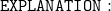• We’re provided – Length , width & height of the rectangular prism are 9 cm , 6 cm & 8 cm respectively & we’re asked to find out the volume of the rectangular prism.
• Remember : The formula to find the volume of the rectangular prism is l × w × h where l is length , w is width & h is height of the rectangular prism.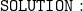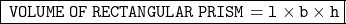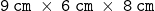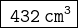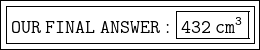▁▁▁▁▁▁▁▁▁▁▁▁▁▁▁▁▁▁▁▁▁▁